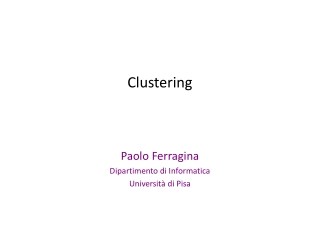DownloadDownload PresentationClustering

# Clustering

Download Presentation## Clustering

- - - - - - - - - - - - - - - - - - - - - - - - - - - E N D - - - - - - - - - - - - - - - - - - - - - - - - - - -
##### Presentation Transcript

1. Clustering Paolo Ferragina Dipartimento di Informatica Università di Pisa

2. Objectives of Cluster Analysis • Finding groups of objects such that the objects in a group will be similar (or related) to one another and different from (or unrelated to) the objects in other groups Competing objectives Intra-cluster distances are minimized Inter-cluster distances are maximized The commonest form of unsupervised learning

3. Notion of similarity/distance • Ideal: semantic similarity • Practical: term-statistical similarity • Docs as vectors • Similarity = cosine similarity, LSH,…

4. Clustering Algorithms • Flat algorithms • Create a set of clusters • Usually start with a random (partial) partitioning • Refine it iteratively • K means clustering • Hierarchical algorithms • Create a hierarchy of clusters (dendogram) • Bottom-up, agglomerative • Top-down, divisive

5. Hard vs. soft clustering • Hard clustering: Each document belongs to exactly one cluster • More common and easier to do • Soft clustering: Each document can belong to more than one cluster. • Makes more sense for applications like creating browsable hierarchies • News is a proper example • Search results is another example

6. Flat & Partitioning Algorithms • Given: a set of n documents and the number K • Find: a partition in K clusters that optimizes the chosen partitioning criterion • Globally optimal • Intractable for many objective functions • Ergo, exhaustively enumerate all partitions • Locally optimal • Effective heuristic methods: K-means and K-medoids algorithms

7. Sec. 16.4 K-Means • Assumes documents are real-valued vectors. • Clusters based on centroids (aka the center of gravity or mean) of points in a cluster, c: • Reassignment of instances to clusters is based on distance to the current cluster centroids.

8. Sec. 16.4 K-Means Algorithm Select K random docs {s1, s2,… sK} as seed centroids. Until clustering converges (or other stopping criterion): For each doc di: Assign di to the cluster crsuch that dist(di, sr) is minimal. For each cluster cj sj = (cj)

9. Sec. 16.4 Pick seeds Reassign clusters Compute centroids Reassign clusters x x Compute centroids x x x x K Means Example (K=2) Reassign clusters Converged!

10. Sec. 16.4 Termination conditions • Several possibilities, e.g., • A fixed number of iterations. • Doc partition unchanged. • Centroid positions don’t change.

11. Sec. 16.4 Time Complexity • The centroids are K • Each doc/centroid consists of M dimensions • Computing distance btw vectors is O(M) time. • Reassigning clusters: Each doc compared with all centroids, O(KNM) time. • Computing centroids: Each doc gets added once to some centroid, O(NM) time. Assume these two steps are each done once for I iterations: O(IKNM).

12. How Many Clusters? • Number of clusters K is given • Partition n docs into predetermined number of clusters • Finding the “right” number of clusters is part of the problem • Can usually take an algorithm for one flavor and convert to the other.

13. Bisecting K-means Variant of K-means that can produce a partitional or a hierarchical clustering SSE = Sum of SquaredError

14. Bisecting K-means Example

15. K-means Pros • Simple • Fast for low dimensional data • Good performance on globular data Cons • K-Means is restricted to data which has the notion of a center (centroid) • K-Means cannot handle non-globular data of different sizes and densities • K-Means will not identify outliers

16. Ch. 17 animal vertebrate invertebrate fish reptile amphib. mammal worm insect crustacean Hierarchical Clustering • Build a tree-based hierarchical taxonomy (dendrogram) from a set of documents • One approach: recursive application of a partitional clustering algorithm

17. Strengths of Hierarchical Clustering • No assumption of any particular number of clusters • Any desired number of clusters can be obtained by ‘cutting’ the dendogram at the proper level

18. Sec. 17.1 Hierarchical Agglomerative Clustering (HAC) • Starts with each doc in a separate cluster • Then repeatedly joins the closest pairof clusters, until there is only one cluster. • The history of mergings forms a binary tree or hierarchy. • The closest pair drives the mergings, how is it defined ?

19. Sec. 17.2 Closest pair of clusters • Single-link • Similarity of the closest points, the most cosine-similar • Complete-link • Similarity of the farthest points, the least cosine-similar • Centroid • Clusters whose centroids are the closest (or most cosine-similar) • Average-link • Clusters whose average distance/cosine between pairs of elements is the smallest

20. p1 p2 p3 p4 p5 . . . p1 p2 p3 p4 p5 . . . How to Define Inter-Cluster Similarity Similarity? • Single link (MIN) • Complete link (MAX) • Centroids • Average Proximity Matrix

21. p1 p2 p3 p4 p5 . . . p1 p2 p3 p4 p5 . . . How to Define Inter-Cluster Similarity • MIN • MAX • Centroids • Average Proximity Matrix

22. p1 p2 p3 p4 p5 . . . p1 p2 p3 p4 p5 . . . How to Define Inter-Cluster Similarity • MIN • MAX • Centroids • Average Proximity Matrix

23. p1 p2 p3 p4 p5 . . . p1 p2 p3 p4 p5 . . . How to define Inter-Cluster Similarity   • MIN • MAX • Centroids • Average Proximity Matrix

24. p1 p2 p3 p4 p5 . . . p1 p2 p3 p4 p5 . . . How to Define Inter-Cluster Similarity • MIN • MAX • Centroids • Average Proximity Matrix